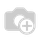# Odoo 10 - Counting values of linked records

In Odoo 10 I have created my own custom application (using the new studio feature), however I have run into an issue trying to compute data between records that belong to different views.

In the scenario I have two models (model A and model B), where records from model B are connect to records from model A via a many2one relational field. There is a field in Model B that counts a numerical value entered into it.

Ideally what I would like to achieve is have some form of Automated Action / Server Action, that loops through the records in Model A, then loops through related records in Model B adding together the values of the previously mentioned numerical value field and sets the value of a field in model A equal to the equated number, before continuing onto the next record.

For example sake say the field names are:

Model A = x_a
- Model A ID Field = x_id_field
- Target field for computed value = x_compute

Model B = x_b
- many2one field = x_a_id
- numerical field = x_value_field

I have attempted to use the automated actions to execute some basic Python code (because I thought this would be as simple as a nested loop) however all my attempts have been failures due to not being familiar with how to loop through records in odoo and how to access other models and their records (from python). How would I go about accomplishing this?